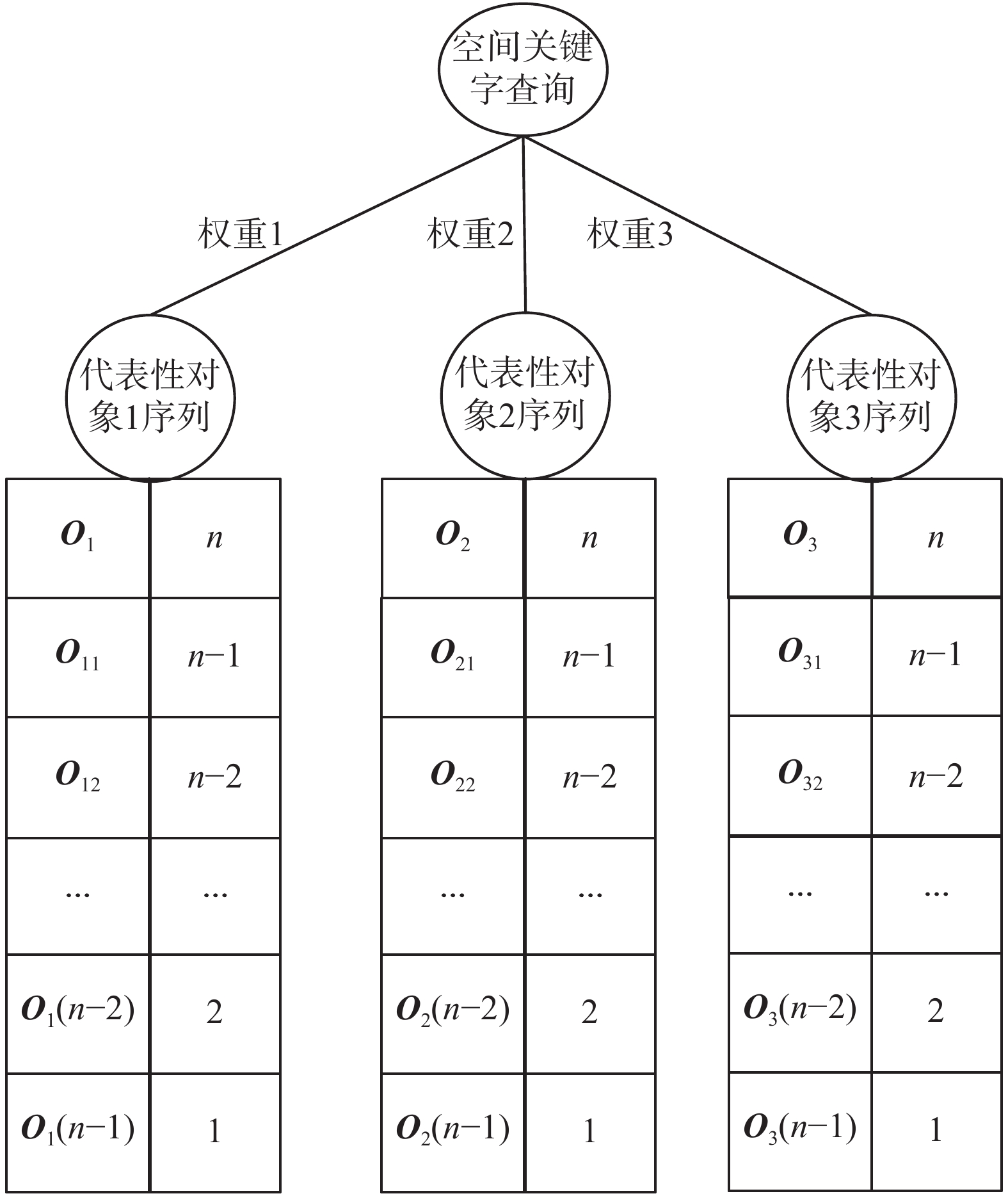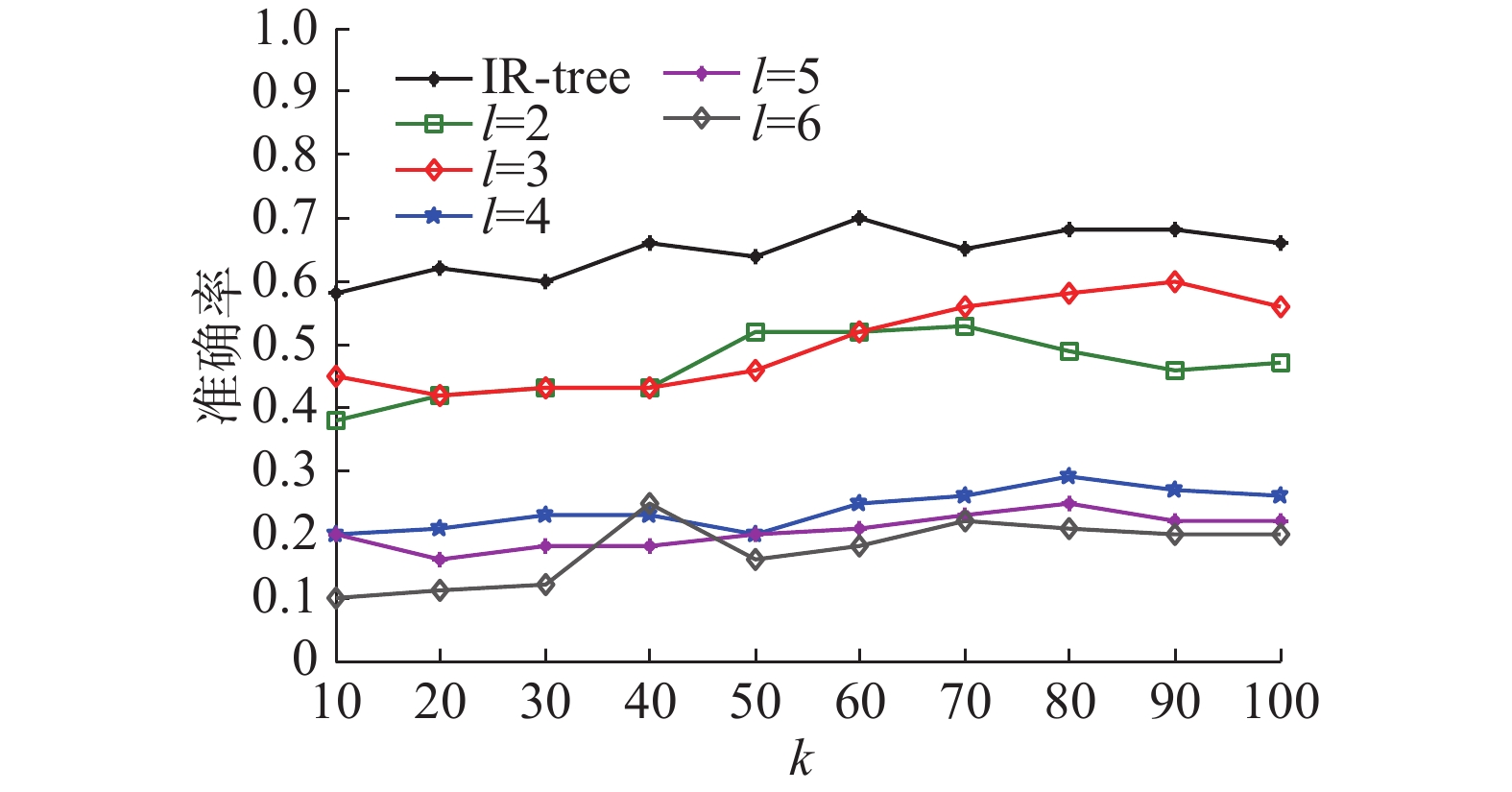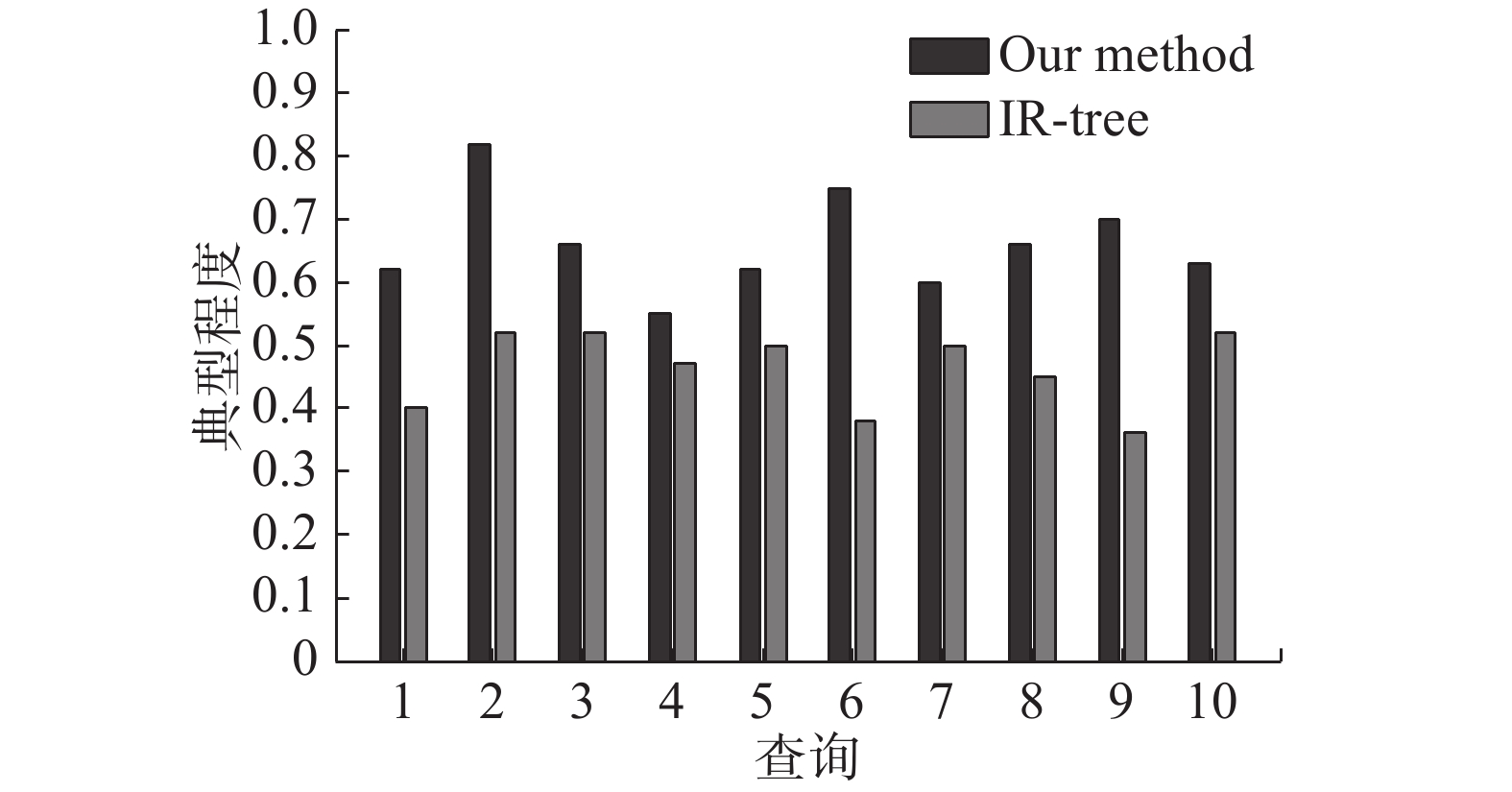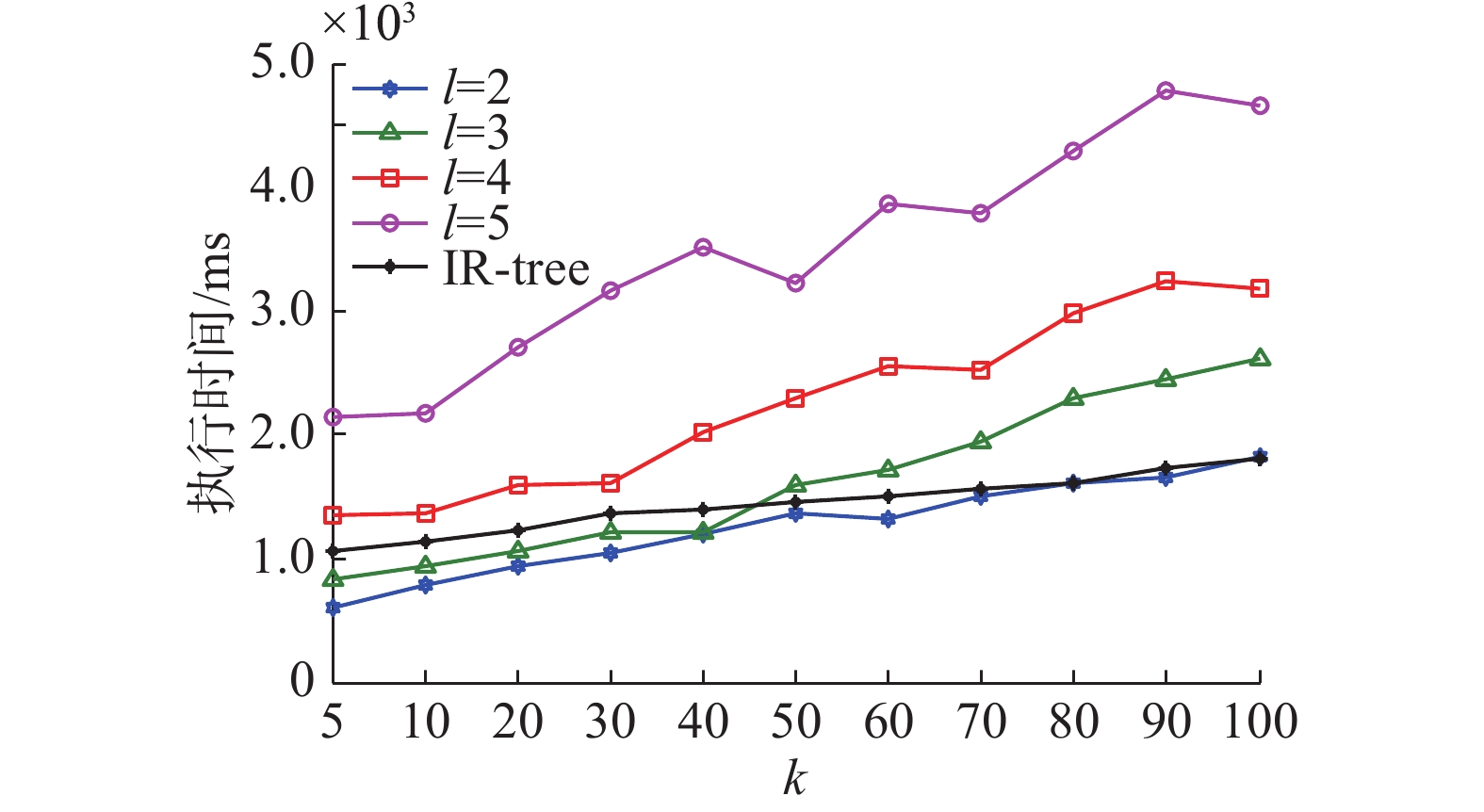﻿ 基于位置−文本关系的空间对象top-<i>k</i>查询与排序方法
«上一篇文章快速检索 高级检索

 智能系统学报2020, Vol. 15Issue (2): 235-242  DOI: 10.11992/tis.2018080110

### 引用本文MENG Xiangfu, ZHANG Xiaoyan, ZHAO Lulu, et al. A location-text correlation-based top-k query and ranking approach for spatial objects [J]. CAAI Transactions on Intelligent Systems, 2020, 15(2): 235-242. DOI: 10.11992/tis.201808011.### 文章历史

A location-text correlation-based top-k query and ranking approach for spatial objects
MENG Xiangfu, ZHANG Xiaoyan , ZHAO Lulu , LI Pan , BI Chongcun
School of Electronic and Information Engineering, Liaoning Technical University, Huludao 125105, China
Abstract: Due to the large size of spatial databases, a common spatial keyword query often leads to the problem of too many answers. To deal with this problem, this paper proposes a location-text correlation-based top- k query and ranking approach for spatial objects, which aims to find the typical spatial objects with high text relevancy and location proximity. This approach consists of offline processing and online query steps. The offline step scores the relationship between any pair of spatial objects by considering their location proximity and text similarity. Then, by using a probabilistic density-based representative spatial object selection method, a set of representatives over the spatial objects is selected to build a corresponding spatial object sequence. In the online query period, when a user issues a spatial keyword query, the location-text correlation between the query and representative objects is evaluated, and then, the top- k typical relevant objects can be expeditiously picked using the threshold algorithm (TA) algorithm over the sequences corresponding to representative spatial objects. The experiments demonstrate that the proposed top- k query and ranking approach can closely meet users' needs, with high precision, typicality, and good performance.
Key words: spatial database; spatial keyword query    location-text correlation    probability density    representative object selection    top-k query and ranking

q: {位置，＜关键字1，关键字 $2, \cdots$ ，关键字m＞}

1 空间对象的位置-文本关系度量表 1 空间数据库实例 Tab.1 An instance of spatial database
1.1 空间对象的位置相近度

 $D({{ o}_i},{{ o}_j}) = \sum\limits_{k = 1}^n {d({ o}_i^{(k)},{ o}_j^{(k)})}$ (1)

 ${\rm Si}{{\rm m}_{{\rm{Loc}}}}({{ o}_i},{{ o}_j}) = 1 - D({{ o}_i},{{ o}_j})/{\rm Max}D$ (2)表 2 空间对象的位置相近度 Tab.2 The location proximity between spatial objects
1.2 空间对象的文本相似度

 $w(t|{ o}.{\rm doc}) = {\rm tf}(t|{ o}.{\rm doc}) * {\rm idf}(t,{ O})$ (3)

 ${\rm Si}{{\rm m}_{{\rm{Doc}}}}({{ o}_{\rm{1}}},{{ o}_{\rm{2}}}){\rm{ = }}\frac{{\displaystyle\sum\limits_{i = 1}^{n} {{{ { o}}_1}[i] {{ { o}}_2}[i]} }}{{\sqrt {\displaystyle\sum\limits_{i = 1}^{n} {{{ { o}}_1}{{[i]}^2}} } *\sqrt {\displaystyle\sum\limits_{i = 1}^{n} {{{ { o}}_2}{{[i]}^2}} } }}$ (4)表 3 空间对象的文本相似度 Tab.3 The text similarity between spatial objects
1.3 空间对象的位置-文本关系紧密度

 ${\rm Sim}({{ o}_i},{{ o}_j}) = \alpha {\rm Si}{{\rm m}_{{\rm{Loc}}}}({{ o}_i},{{ o}_j}) + (1 - \alpha ) {\rm Si}{{\rm m}_{{\rm{Doc}}}}({{ o}_i},{{ o}_j})$ (5)表 4 空间对象的位置-文本关系紧密度 Tab.4 The location-text closeness between spatial objects

2 top-k结果选取与排序方法

q代表一个空间关键字查询，O是空间对象集合，查询结果的top-k选取与排序问题定义如下：

 ${\Gamma _k} = \arg {\max _\Gamma }\sum\limits_{i = 1}^{k(k \ll n)} {{\rm Sim}(q,{{ o}_i})}$ (6)

2.1 选取代表性空间对象

 $f({{ o}_i}) = \frac{1}{n}\sum\limits_{j = 1}^n {{G_h}({{ o}_i},{{ o}_j})} = \frac{1}{{n\sqrt {2{\text{π}} } }}\sum\limits_{j = 1}^n {{{\rm{e}}^{ - \frac{{{\rm{d}}{{({{ o}_i},{{ o}_j})}^2}}}{{2{h^2}}}}}}$ (7)

1)将空间对象集合O随机划分成若干小组，每个小组都包含u个空间对象，即将集合O划分成了n/u个小组，接着在每个小组中利用式(7)计算所有空间对象的概率密度，选取每个小组中概率密度最高的对象构成一个集合，然后从O中将其他对象去除。

2)对于新得到的集合，重复上述过程，直到空间对象集合O中只剩下一个对象为止，并将该对象放入代表性对象候选集合中(上述过程记为一次选取过程)。

3)为了保证选取对象的准确性，需要将上述选取过程重复执行m次(记为一轮)，这样代表性对象候选集合中最多会包含m个空间对象，接着在最初的空间对象集合O上计算这m个对象的概率密度，最后将具有最高概率密度的对象作为当前轮次的选取结果，并从O中将该空间对象去除。上述整个过程重复l轮，这样就能得到l个近似于准确解的代表性空间对象。

2.2 创建空间对象序列

 $s({{ o}_i}|\tau ) = n - p({{ o}_i}) + 1$ (8)Download: 图 1 代表性空间对象序列示意 Fig. 1 Orders corresponding to representative objects

2.3 top-k结果选取与排序

 ${\rm{score(}}{ q},{{ o}_j}{\rm{)}} = \sum\limits_{i = 1}^l {{\rm Sim}({ q},{{\bar { o}}_i}) s({{ o}_j}|{{\tau} _i})}$ (9)

1) 令B={ }是一个缓存

2) 令L是一个大小为l的数组，存储每个序列中最近一次检索得到的分数(score)

3) repeat

4) for each $i \in \left\{ {1,{\rm{ }}2, \cdots ,l} \right\}$ do

5) 从序列 ${{ \tau} _i}$ 中检索下一个对象oj，计算oj ${{\tau} _i}$ 中的分数： $s({{ o}_j},q) = {\rm Sim}({ q},{\bar { o}_i}) s({{ q}_j}|{{\tau} _i})$

6) 用对象oj ${\tau _i}$ 中的分数更新L中对应于对象oj的分数

7) 利用随机访问方式获取oj在其他序列中的分数，将所有检索到的分数加权求和，得到score(oj,q)

8) 按照降序方式，将<oj, score(oj,q)>插入到B中的正确位置

9) end for

10) until $B\left[ k \right]{\rm score} \geqslant \sum\limits_{i = 1}^l {L[i]}$

11) return B

1)循环访问每个代表性序列。在每次循环过程中，当一个空间对象oj在某个序列 ${{\tau} _{{i}}}$ 中被发现时，计算它在该代表性序列中的分数，计算公式为

 $s({{ o}_j},{ q}) = {\rm Sim}({ q},{\bar { o}_i}) s({{ o}_j}|{{\tau} _i})$ (10)

2)令 $s({{ o}_m}|{{\tau} _i})$ 为第m次循环结束后，在每个序列 ${{\tau} _{{i}}}$ 中最后被访问对象的分数。TA算法阈值threshold为第j次循环结束后数组L中的分数之和。

 ${\rm threshold} = \sum\limits_{i = 1}^l {{\rm Sim}({ q},{{\bar { o}}_i})} s({{ o}_m}|{{ \tau} _i})$ (11)

3)在所有被发现的空间对象中，输出前k个具有最高总体排序分数的对象。

3 性能实验分析 3.1 实验环境表 5 测试数据集特点 Tab.5 The properties of Yelp dataset
3.2 top-k结果的准确性和典型性测试

 ${\rm{J}}(R({\rm Rep},k),R({\rm All},k)){\rm{ = }}\frac{{\left| {R({\rm Rep},k) \cap R({\rm All},k)} \right|}}{{\left| {R({\rm Rep},k) \cup R({\rm All},k)} \right|}}$ (12)

Jaccard系数的值在[0,1]之间，值越高表明2个集合的重叠度越高，即top-k结果的准确性也就越高。Download: 图 2 利用本文方法和IR-tree索引得到的top-k结果准确性对比 Fig. 2 Comparison for the precision of top-k results returned by using our method and IR-tree index

 ${\rm Typicality}(T) = \frac{{\displaystyle\sum\limits_{i = 1}^k {f({o_i})} }}{k}$ (13)Download: 图 3 本文方法和IR-tree得到的top-k结果典型程度对比 Fig. 3 Comparison for the typicality of the top-k results returned by using our method and IR-tree index

3.3 top-k查询算法的性能测试Download: 图 4 不同l和k值下利用本文方法和IR-tree的top-k查询响应时间 Fig. 4 Execution time of top-k query selection by using our method and IR-tree index under different values of l and k

4 结束语

  QI Jianzhong, ZHANG Rui, JENSEN C S. Continuous spatial query processing: a survey of safe region based techniques[J]. ACM computing surveys, 2018, 51(3): 64. (0)  CONG Gao, JENSEN C S. Querying geo-textual data: spatial keyword queries and beyond[C]//Proceedings of 2016 International Conference on Management of Data. San Francisco, California, USA, 2016: 2207−2212. (0)  ZHENG Kai, SU Han, ZHENG Bolong, et al. Interactive top-k spatial keyword queries[C]//Proceedings of the 31st International Conference on Data Engineering. Seoul, South Korea, 2015: 423–434. (0)  LU Ying, LU Jiaheng, CONG Gao, et al. Efficient algorithms and cost models for reverse spatial-keyword k-nearest neighbor search [J]. ACM transactions on database systems, 2014, 39(2): 13. (0)  DE FELIPE I, HRISTIDIS V, RISHE N. Keyword search on spatial databases[C]//Proceedings of the 24th IEEE International Conference on Data Engineering. Cancun, Mexico, 2008: 656–665. (0)  LI Guoliang, XU Jing, FENG Jianhua. Keyword-based k-nearest neighbor search in spatial databases[C]//Proceedings of the 21st ACM International Conference on Information and Knowledge Management. Maui, Hawaii, USA, 2012: 2144–2148. (0)  WANG Xiang, ZHANG Ying, ZHANG Wenjie, et al. Skype: top-k spatial-keyword publish/subscribe over sliding window[J]. Proceedings of the VLDB endowment, 2016, 9(7): 588-599. DOI:10.14778/2904483.2904490 (0)  ZHANG Dongxiang, CHEE Y M, MONDAL A, et al. Keyword search in spatial databases: towards searching by document[C]//Proceedings of the 25th International Conference on Data Engineering. Shanghai, China, 2009: 688–699. (0)  CONG Gao, JENSEN C S, WU Dingming. Efficient retrieval of the top-k most relevant spatial web objects[J]. Proceedings of the VLDB endowment, 2009, 2(1): 337-348. DOI:10.14778/1687627.1687666 (0)  CHEN Lei, LIN Xin, HU Haibo, et al. Answering why-not questions on spatial keyword top-k queries[C]//Proceedings of the 31st International Conference on Data Engineering. Seoul, South Korea, 2015: 279–290. (0)  KWON H Y, WANG Haixun, WHANG K Y. G-index model: a generic model of index schemes for top-k spatial-keyword queries[J]. World wide web, 2015, 18(4): 969-995. DOI:10.1007/s11280-014-0294-0 (0)  GUTTMAN A. R-trees: a dynamic index structure for spatial searching[C]//Proceedings of 1984 ACM SIGMOD International Conference on Management of Data. Boston, Massachusetts, 1984: 47–57. (0)  BECKMANN N, KRIEGEL H P, SCHNEIDE R, et al. The R*-tree: an efficient and robust access method for points and rectangles[C]//Proceedings of 1990 ACM SIGMOD International Conference on Management of Data. Atlantic City, New Jersey, USA, 1990: 322–331. (0)  ZHANG Chengyuan, ZHANG Ying, ZHANG Wenjie, et al. Inverted linear quadtree: efficient top k spatial keyword search[J]. IEEE transactions on knowledge and data engineering, 2016, 28(7): 1706-1721. DOI:10.1109/TKDE.2016.2530060 (0)  ZHOU Yinghua, XIE Xing, WANG Chuang, et al. Hybrid index structures for location-based Web search[C]//Proceedings of the 14th ACM International Conference on Information and Knowledge Management. Bremen, Germany, 2005: 155–162. (0)  LI Zhisheng, LEE K C K, ZHENG Baihua, et al. IR-Tree: an efficient index for geographic document search[J]. IEEE transactions on knowledge and data engineering, 2011, 23(4): 585-599. DOI:10.1109/TKDE.2010.149 (0)  HONG H J, CHIU G M, TSAI W Y. A single quadtree-based algorithm for top-k spatial keyword query[J]. Pervasive and mobile computing, 2017, 42: 93-107. DOI:10.1016/j.pmcj.2017.09.009 (0)  HUA Ming, PEI Jian, FU A W C, et al. Top-k typicality queries and efficient query answering methods on large databases [J]. The VLDB journal, 2009, 18(3): 809-835. DOI:10.1007/s00778-008-0128-8 (0)  FAGIN R, LOTEM A, NAOR M. Optimal aggregation algorithms for middleware[J]. Journal of computer and system sciences, 2003, 66(4): 614-656. DOI:10.1016/S0022-0000(03)00026-6 (0)